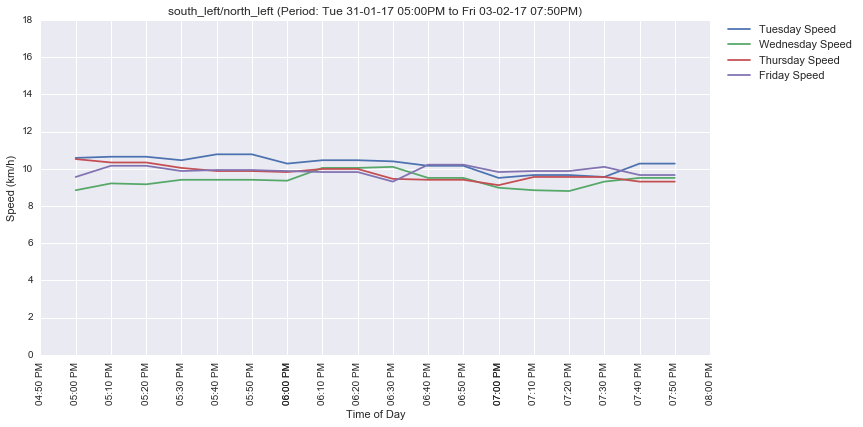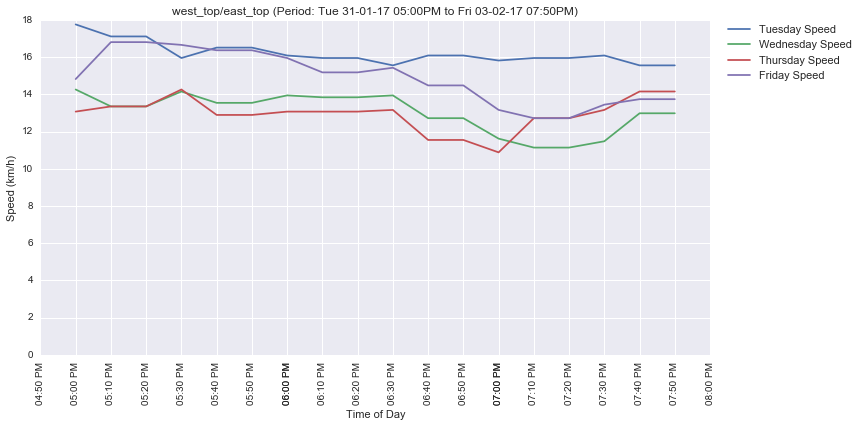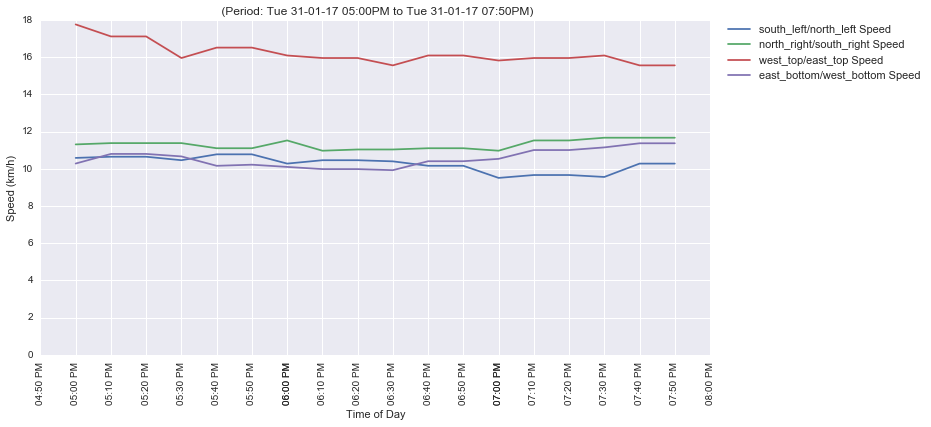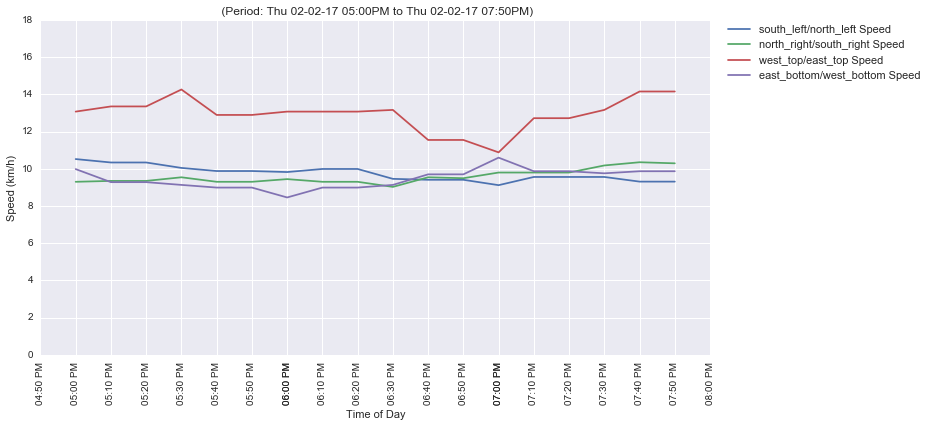In :
import matplotlib.pyplot as plt
import seaborn as sb
import mh_db as db
import mh_lib as ml
from IPython.display import display, HTML
import datetime
import pandas as pd
import numpy as np
%matplotlib inline

In :
gmaps = ml.gmaps()

In :
path_id=5
signal_coords = db.get_paths([path_id])['coordinates']
segment_coords = ml.get_signal_segments_coords(path_id, signal_coords)
#ml.run_signal_capture(gmaps, segment_coords, year=2017, month=2, day=6, start_hour=17, end_hour=20, csv='csv/signal_data.csv')

In :
HTML(data=ml.show_signal_map(path_id))

Traffic signal analysis: Hope Farm Junction

Out:
In :
print("Traffic junction model")
HTML(data='<img src="https://traffic.mapshalli.org/reports/Traffic_Junction_Model.jpg"></img>')

Traffic junction model

Out:In :
df = ml.read_signal_csv('csv/signal_data.csv')

In :
for label in ['south_left/north_left', 'west_top/east_top']:
df_f = df[df.name == label]
df_g = df_f.groupby(df_f.date.dt.dayofweek).mean()
ml.plot_signal_traffic(plt, df_f, by_dow=True, title=label)In :
ml.plot_signal_traffic(plt, df[df.date.dt.dayofweek==ml.DOW['TUE']], by_labels=True, labels=['south_left/north_left', 'north_right/south_right', 'west_top/east_top', 'east_bottom/west_bottom'])In :
ml.plot_signal_traffic(plt, df[df.date.dt.dayofweek==ml.DOW['THU']], by_labels=True, labels=['south_left/north_left', 'north_right/south_right', 'west_top/east_top', 'east_bottom/west_bottom'])